From the sum of and subtract

# From the sum of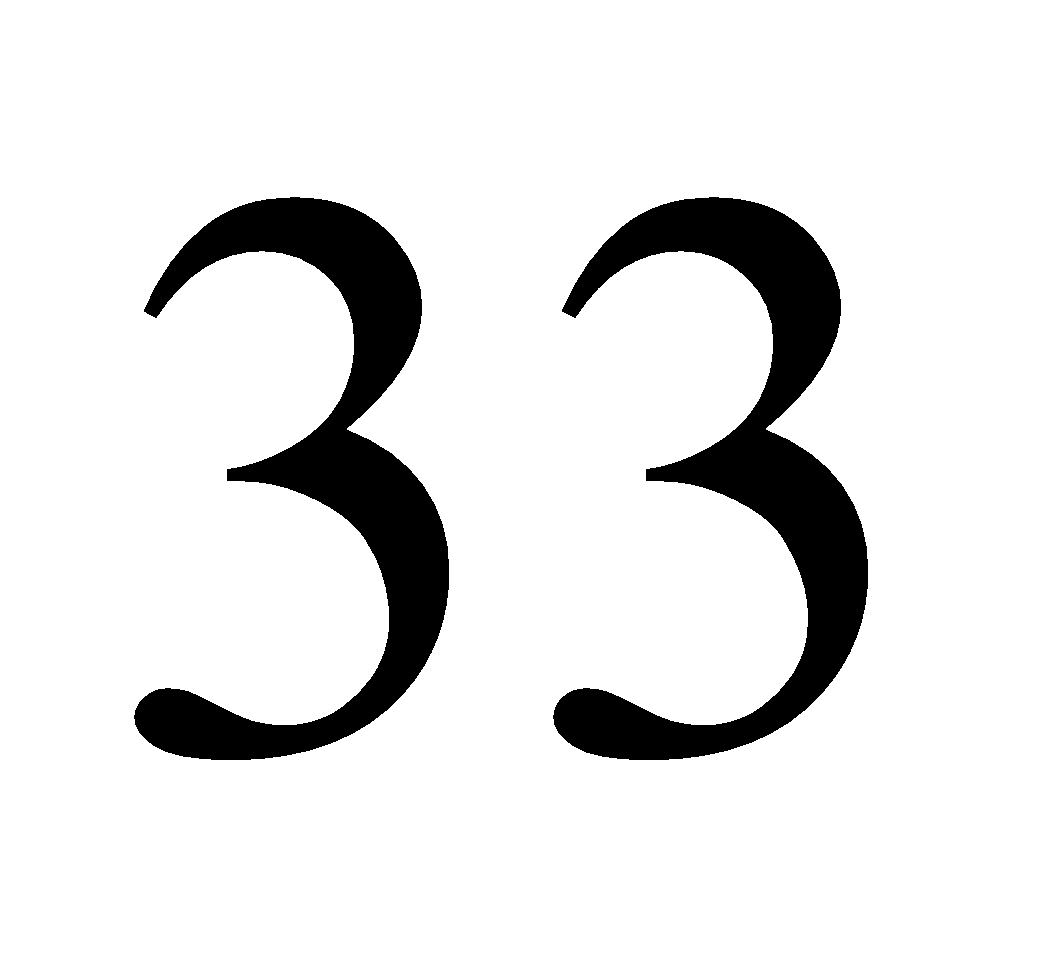and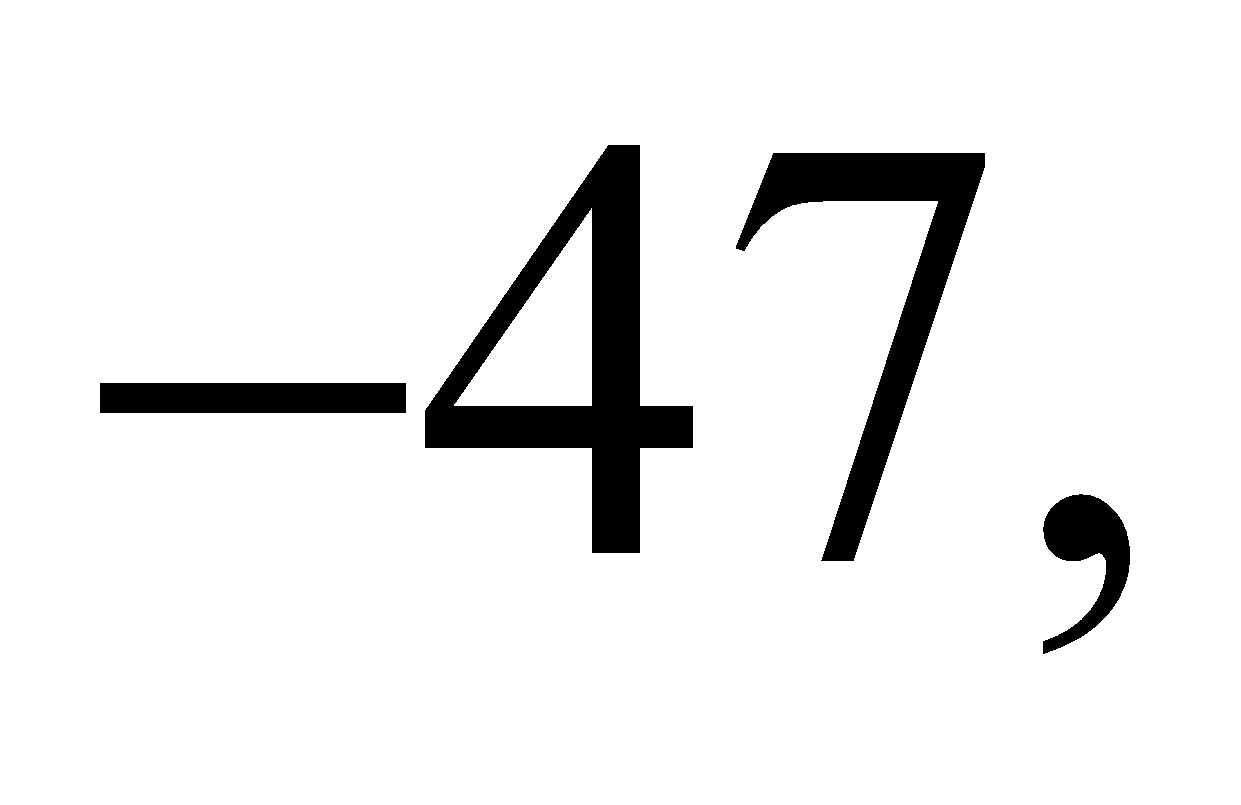subtract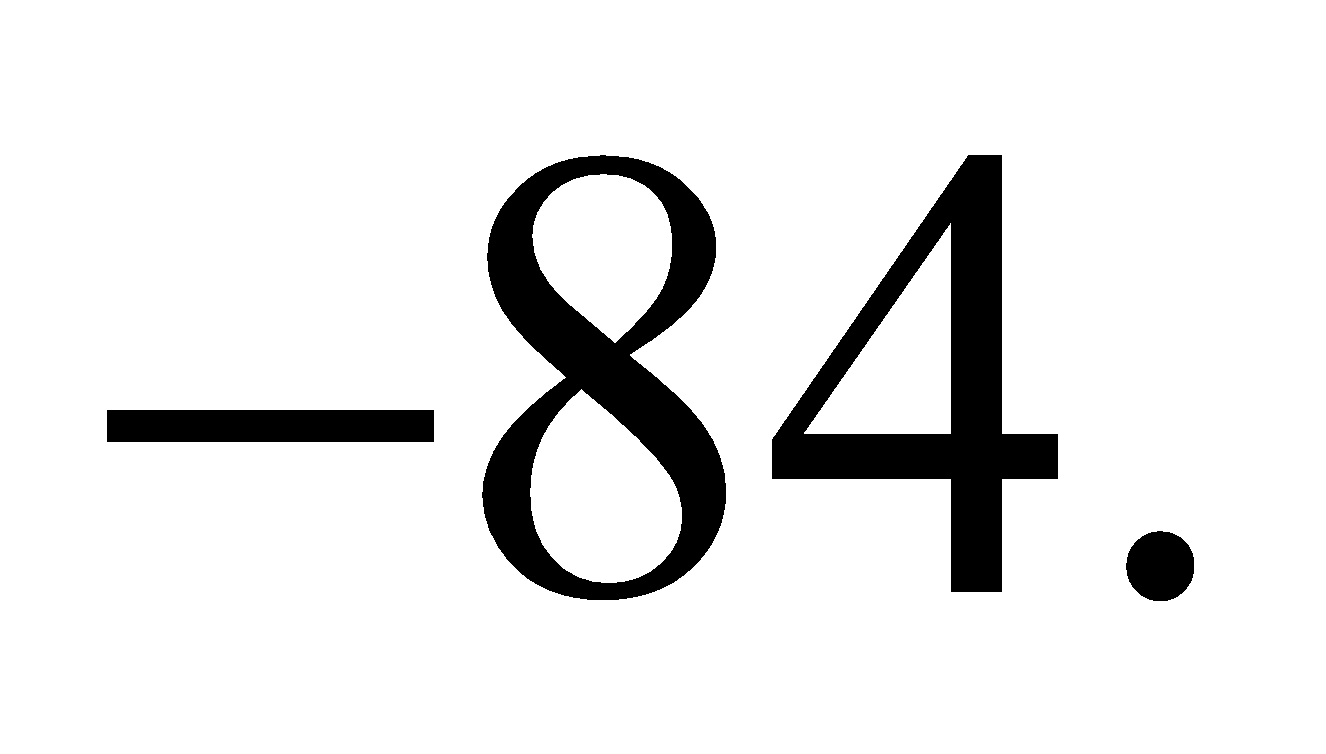1. A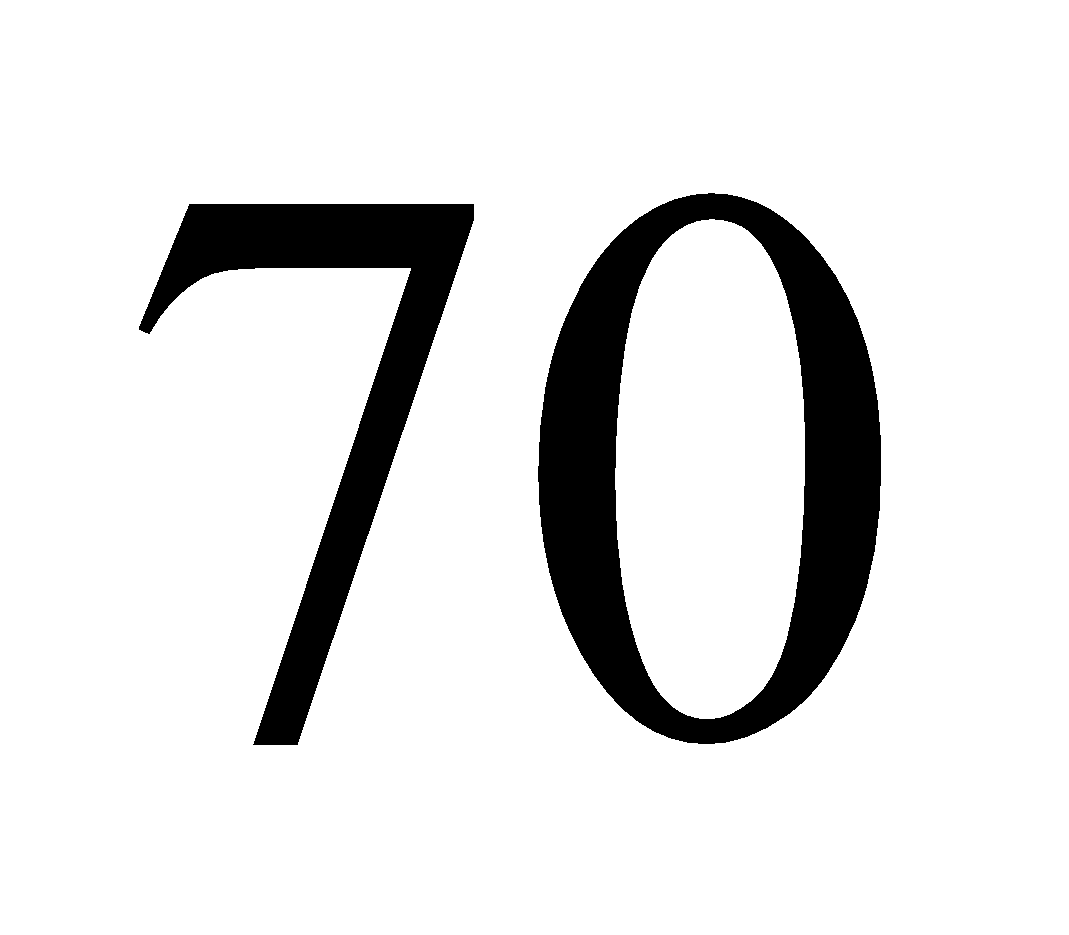2. B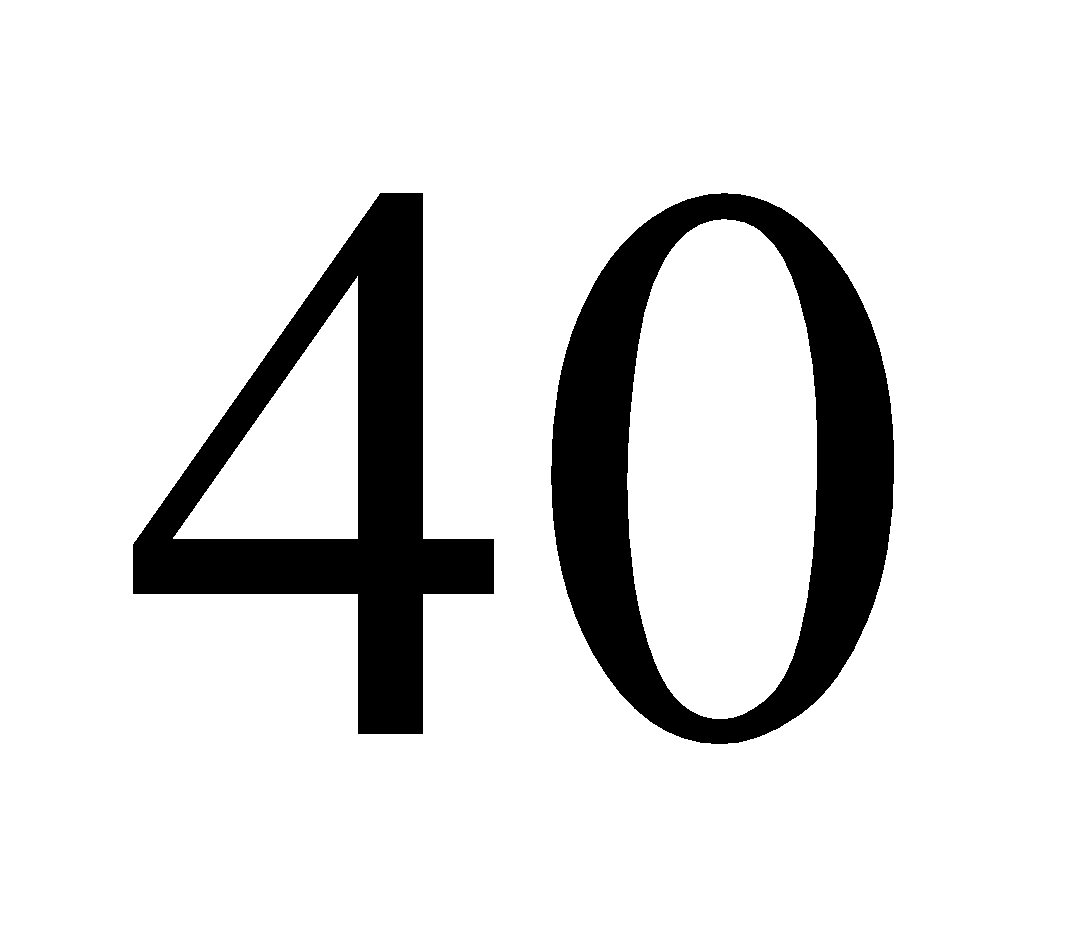3. C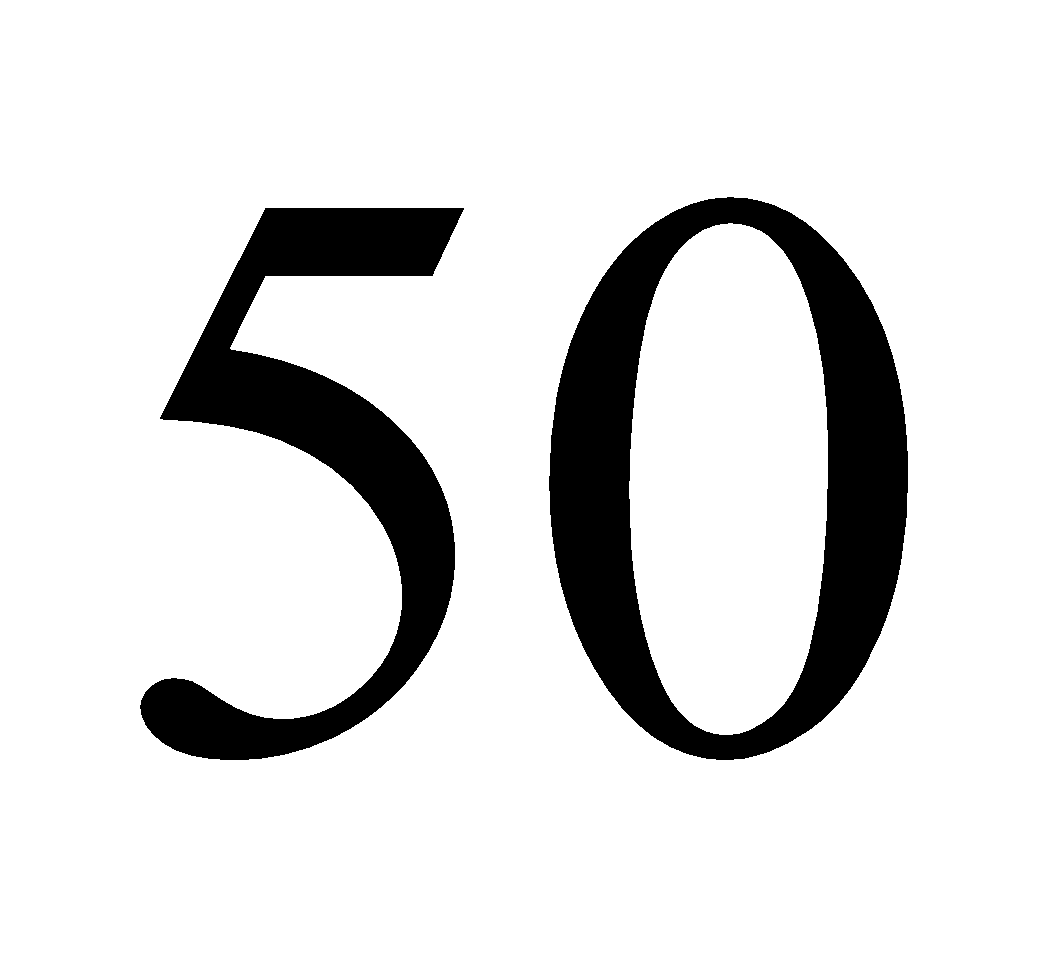4. D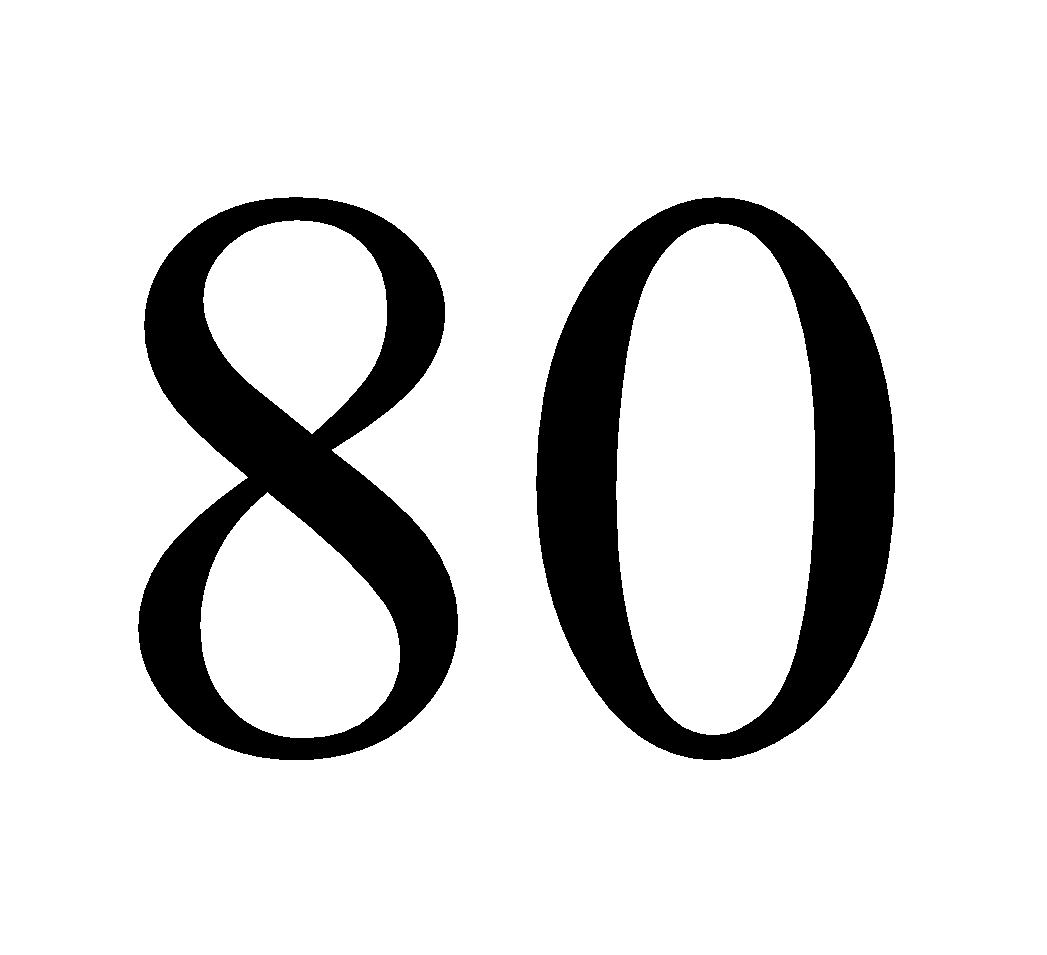Fill Out the Form for Expert Academic Guidance!l

+91

Live ClassesBooksTest SeriesSelf Learning

Verify OTP Code (required)

### Solution:

Concept: In this question, students need to pay attention to the signs along with the numbers. Let's start with a total of 33 to solve the question. And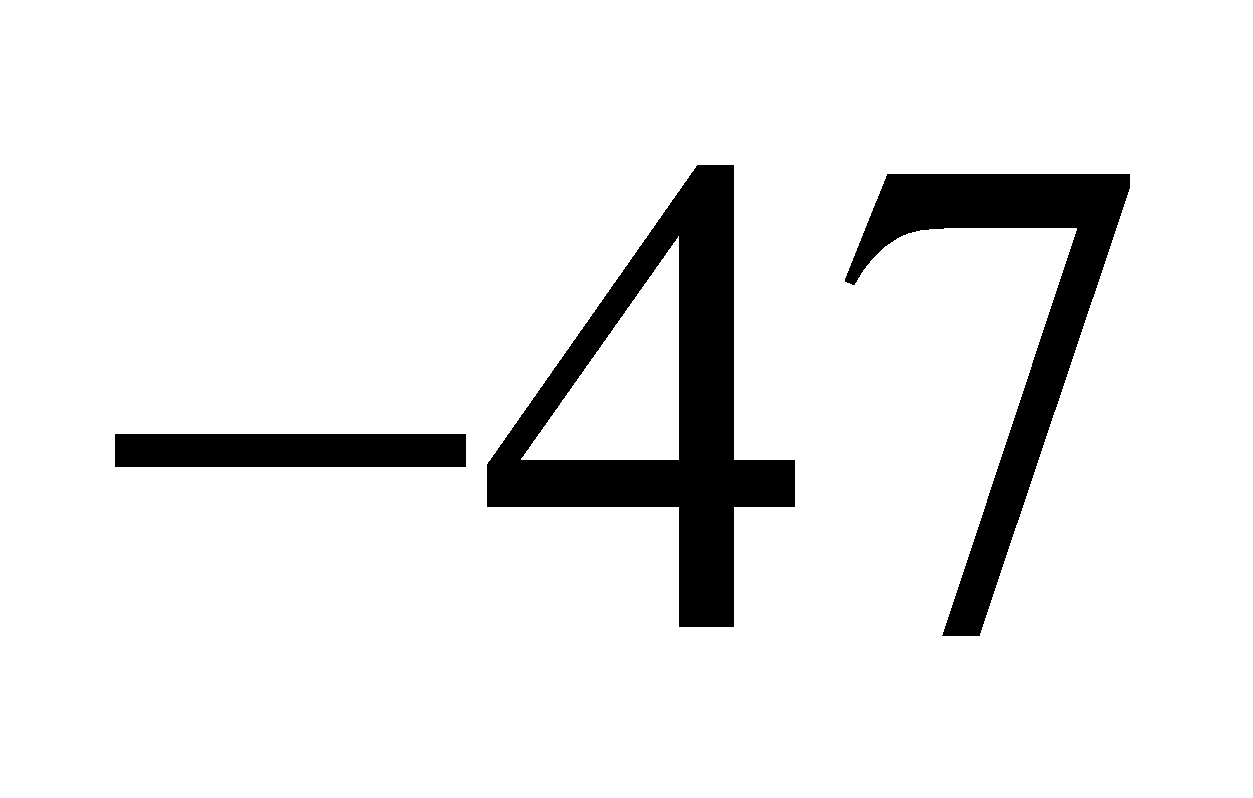,  So the answer is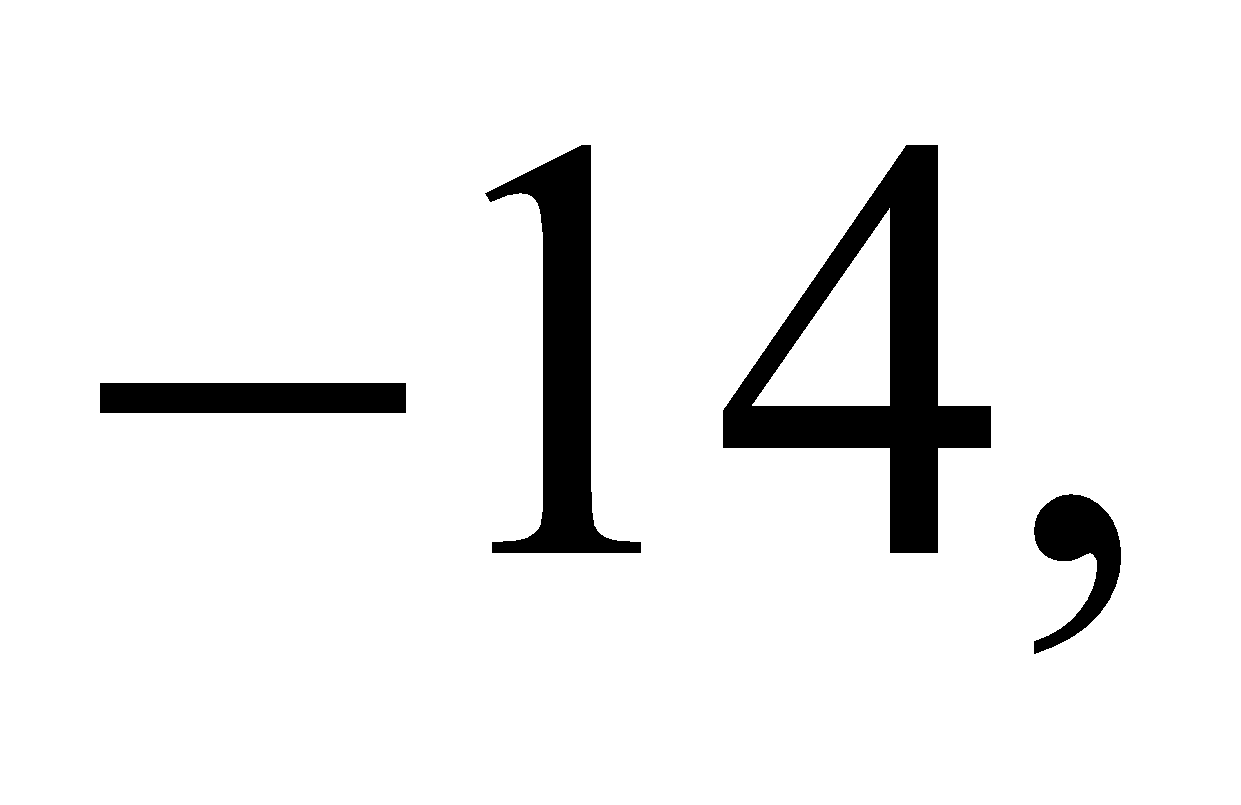then subtractFrom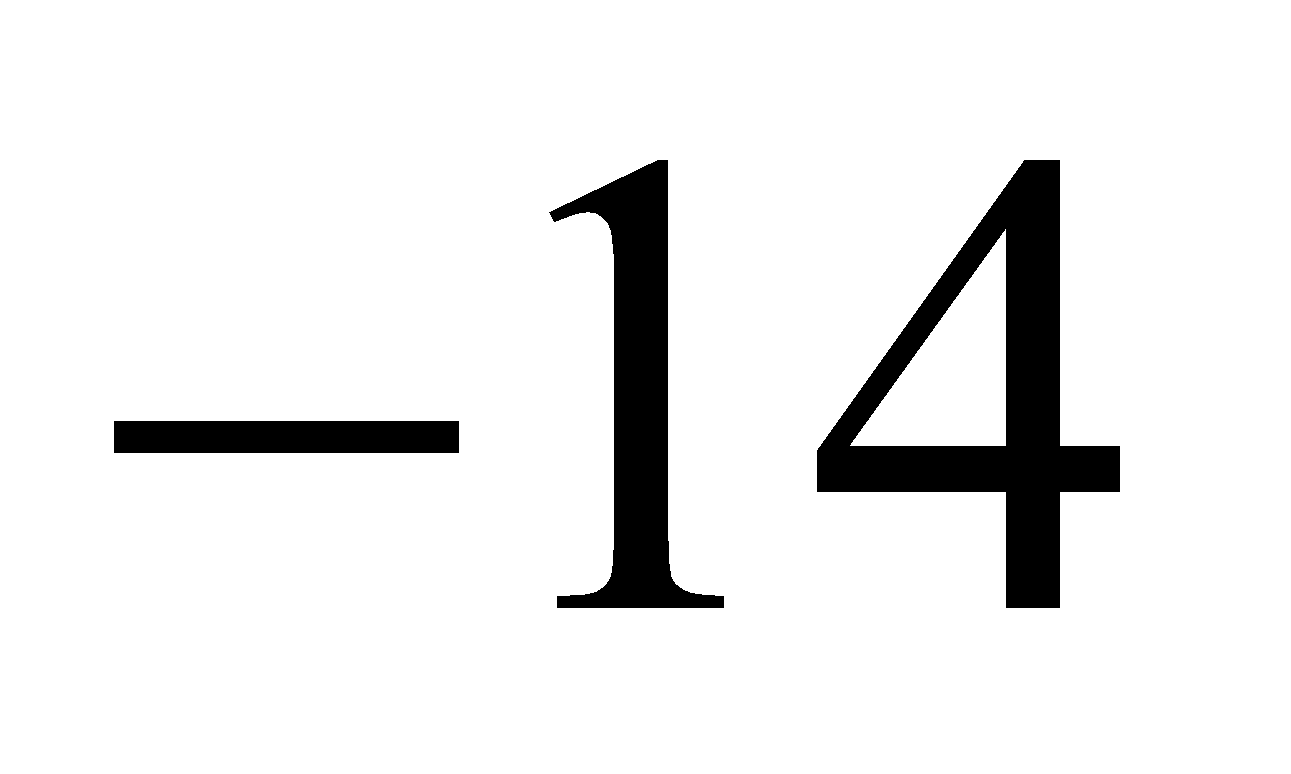And you get the response you want.
From the sum of 33 and, subtract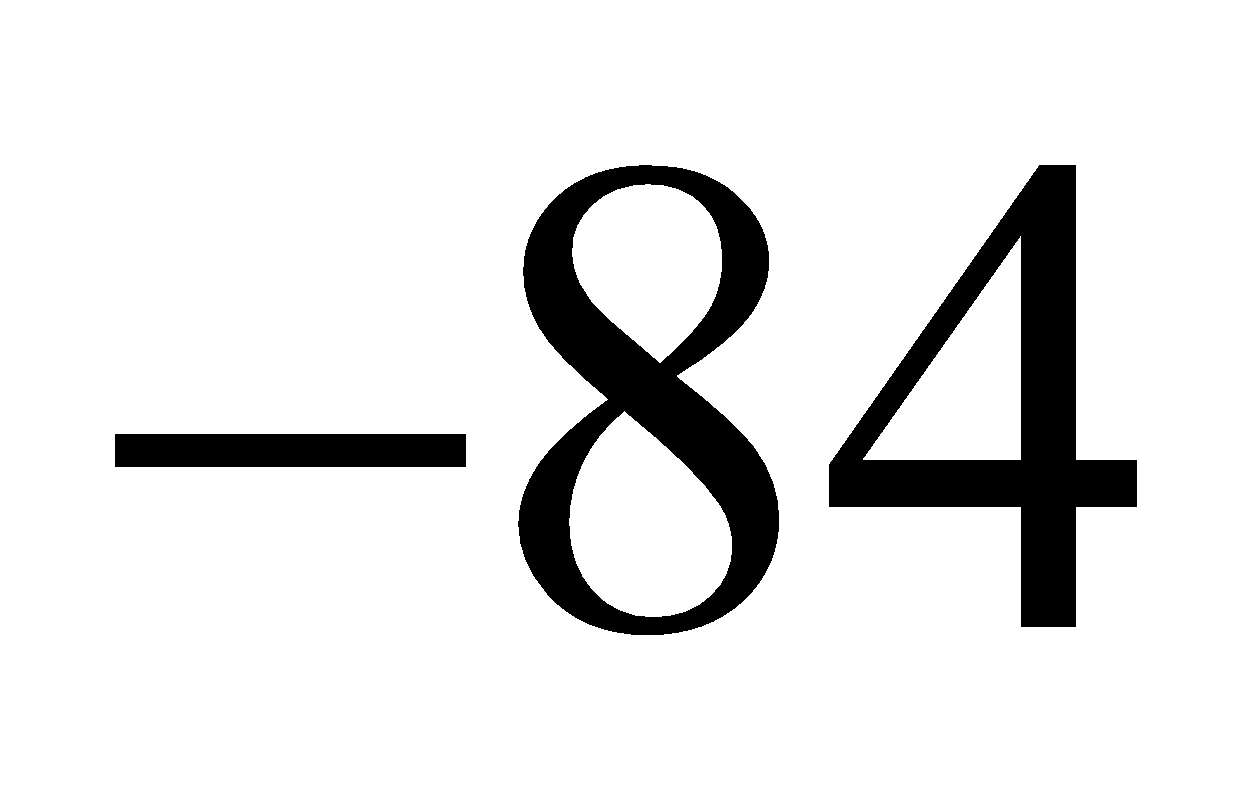.
So, by the language of question we write the integers like this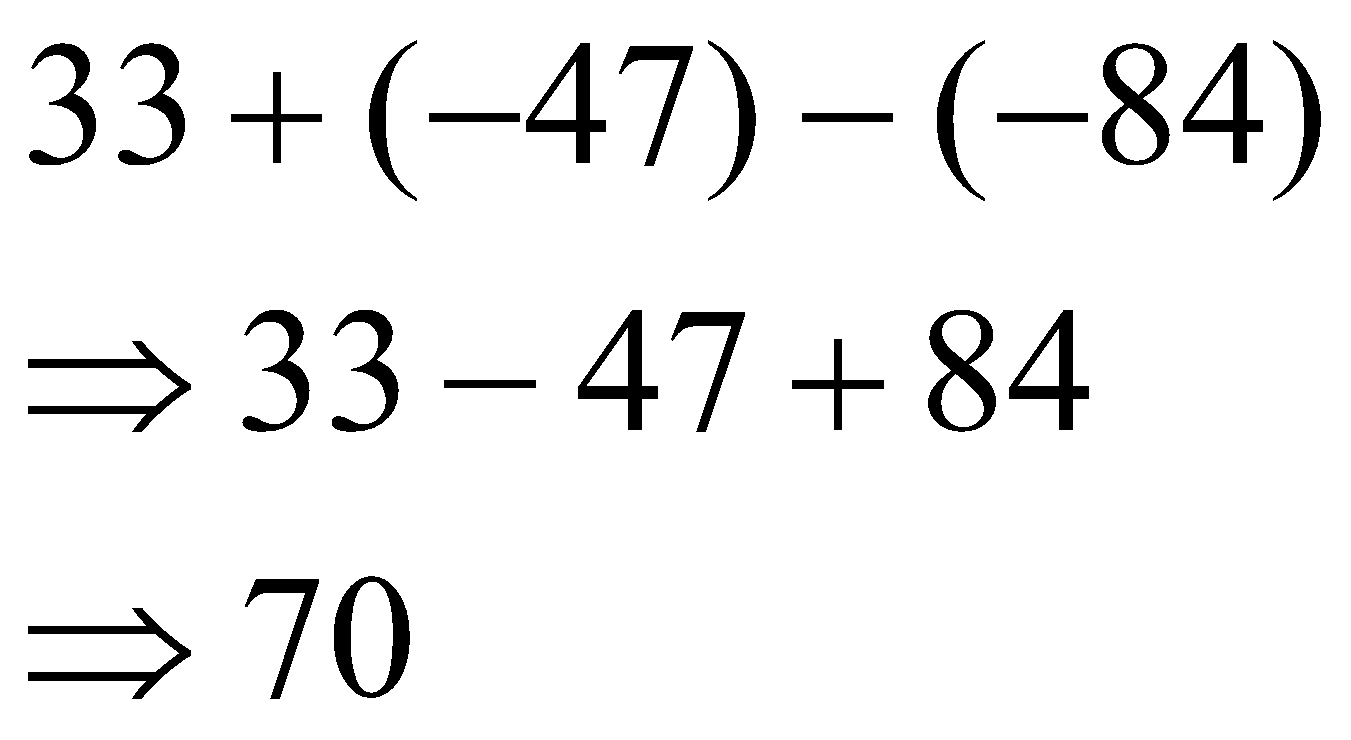Hence, option 1 is correct.

## Related content

 Area of Square Area of Isosceles Triangle Pythagoras Theorem Triangle Formula Perimeter of Triangle Formula Area Formulae Volume of Cone Formula Matrices and Determinants_mathematics Critical Points Solved Examples Type of relations_mathematics+91

Live ClassesBooksTest SeriesSelf Learning

Verify OTP Code (required)